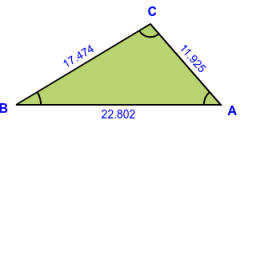# Solve 13

Solve the missing dimensions for the following triangle: Triangle ABC: AngleA=43 degrees, b=7.0cm, c=6.0cm

Question 1. Angle B with units written as degrees
Question 2. Angle C with units written as degrees
Question 3. a, rounded to the nearest tenth of a cm with the unit.

a =  4.8545 cm
B =  79.5497 °
C =  57.4503 °

### Step-by-step explanation:

Try calculation via our triangle calculator.Did you find an error or inaccuracy? Feel free to write us. Thank you!

Tips for related online calculators
Need help calculating sum, simplifying, or multiplying fractions? Try our fraction calculator.
Do you want to convert length units?
The Pythagorean theorem is the base for the right triangle calculator.
Do you want to convert time units like minutes to seconds?
Cosine rule uses trigonometric SAS triangle calculator.